EnchantedLearning.com is a user-supported site.
As a bonus, site members have access to a banner-ad-free version of the site, with print-friendly pages.

 You might also like: Multiplying By Two, Part 2 - Early Multiplication Multiplying By Three, Part 2 - Early Multiplication Multiplying By Five, Part 2 - Early Multiplication Multiplying By Six, Part 2 - Early Multiplication Adding Groups of Numbers #2 - Early Multiplication Today's featured page: VENUS' Atmosphere

 More Math Activities EnchantedLearning.comEarly Multiplication Printouts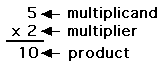More Multiplication Activities

The following are multiplication printouts.

 2, 4, 6, 8, 10, ...Skip CountingPrintablesSkip counting is good addition practice and a great introduction to multiplication. Count by 2s, 3s, s, and so on in these worksheets.Early MultiplicationAdding Groups of Numbers #1Add bowls of eggs and plates of cookies to develop the idea of multiplication as the addition of groups of numbers. Or go to the answers.Early MultiplicationAdding Groups of Numbers #2Add apples on trees and boxes of oranges to develop the idea of multiplication as the addition of groups of numbers. Or go to the answers.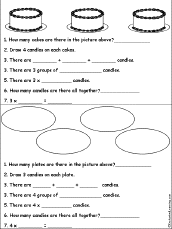Early MultiplicationAdding Groups of Numbers #3Add candles on cakes and plates of candies to develop the idea of multiplication as the addition of groups of numbers. Or go to the answers.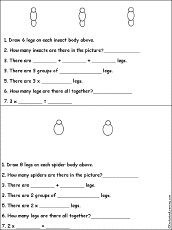Early MultiplicationAdding Groups of Numbers #4Add legs on insects and spiders to develop the idea of multiplication as the addition of groups of numbers. Or go to the answers.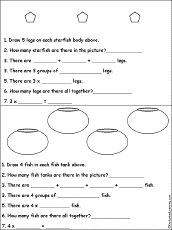Early MultiplicationAdding Groups of Numbers #5Add legs on starfish and fish in tanks to develop the idea of multiplication as the addition of groups of numbers. Or go to the answers.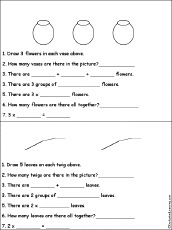Early MultiplicationAdding Groups of Numbers #6Add flowers in vases and leaves on twigs to develop the idea of multiplication as the addition of groups of numbers. Or go to the answers.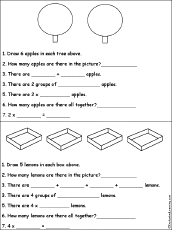Early MultiplicationAdding Groups of Numbers #7Add apples in trees and lemons in boxes to develop the idea of multiplication as the addition of groups of numbers. Or go to the answers.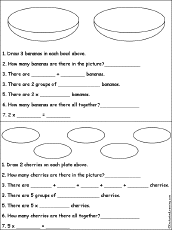Early MultiplicationAdding Groups of Numbers #8Add bananas in bowls and cherries on plates to develop the idea of multiplication as the addition of groups of numbers. Or go to the answers.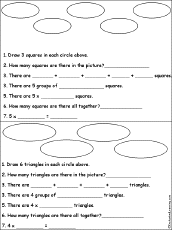Early MultiplicationAdding Groups of Numbers #9Add groups of squares and triangles to develop the idea of multiplication as the addition of groups of numbers. Or go to the answers.Early MultiplicationDraw Groups of Shapes #1Draw groups of shapes and write equations that describe them. For example, "Draw 2 groups of 3 circles. 2 x 2 = 4" Or go to the answers.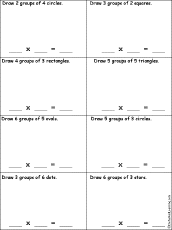Early MultiplicationDraw Groups of Shapes #2Draw groups of shapes and write equations that describe them. For example, "Draw 3 groups of 6 dots. 3 x 6 = 18" Or go to the answers.Early MultiplicationDraw Groups of Shapes #3Draw groups of shapes and write equations that describe them. For example, "Draw 5 groups of 2 triangles. 3 x 6 = 18" Or go to the answers.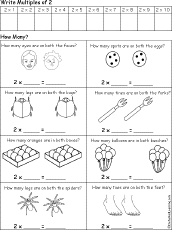Early Multiplication: Count by 2s Count by twos (2 x 1 through 2 x 10) and then write how many objects are in two groups, for example, "How many legs are on both bugs?" Or go to the answers.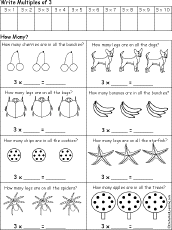Early Multiplication: Count by 3s Count by threes (3 x 1 through 3 x 10) and then write how many objects are in three groups, for example, "How many legs are on all the dogs?" Or go to the answers.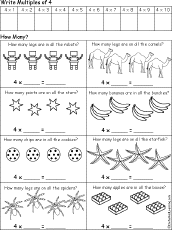Early Multiplication: Count by 4s Count by fours (4 x 1 through 4 x 10) and then write how many objects are in four groups, for example, "How many points are on all the stars?" Or go to the answers.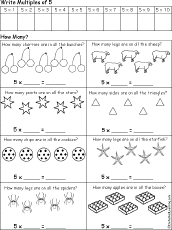Early Multiplication: Count by 5s Count by fives (5 x 1 through 5 x 10) and then write how many objects are in five groups, for example, "How many sides are on all the triangles?" Or go to the answers.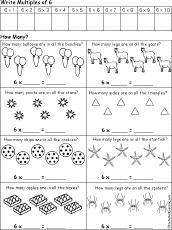Early Multiplication: Count by 6s Count by sixes (6 x 1 through 6 x 10) and then write how many objects are in six groups, for example, "How many chips are in all the cookies?" Or go to the answers.Early Multiplication: Count by 7s Count by sevens (7 x 1 through 7 x 10) and then write how many objects are in seven groups, for example, "How many fingers are in all the gloves?" Or go to the answers.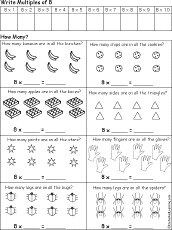Early Multiplication: Count by 8s Count by eights (8 x 1 through 8 x 10) and then write how many objects are in eight groups, for example, "How many bananas are in all the bunches?" Or go to the answers.Early Multiplication: Count by 9s Count by nines (9 x 1 through 9 x 10) and then write how many objects are in nine groups, for example, "How many sides are on all the squares?" Or go to the answers.Animal Arithmetic (with pictures)A Printable Activity BookA short, printable book about addition for early readers. The book has pages for the student to cut out, questions to read, pictures to help solve the questions (like, "How many legs do 2 ladybugs have?"), and objects to count. The pages include: tiger tails, eagle eyes, toad tongues, bird beaks, wasp wings, fox feet, snail shells, elephant ears, shark snouts, ladybug legs, antelope antlers, ant antennae, and moose mouths.Animal Arithmetic (no pictures)A Printable Activity BookA short, printable book about addition for early readers. The book has pages for the student to cut out, questions to read, pictures to draw in order to solve the questions (like, "How many paws do 3 cats have?"), and objects to count. The pages include: bird wings, elephant trunks, dog eyes, spider legs, monkey tails, rabbit ears, octopus arms, bull horns, people toes, cat paws, and frog mouths.Multiplication: Match Groups of NumbersMatch groups of numbers, for example, match "2 groups of 3"to "2 x 3" to "2 threes." Or go to the answers.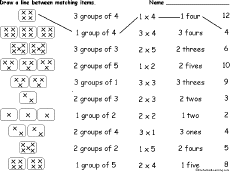Multiplication: Match Groups of Numbers #2Match groups of numbers, for example, match "2 groups of 3"to "2 x 3" to "2 threes." Or go to the answers. Multiplication Table 1-12Print a multiplication table of the numbers 1 to 12.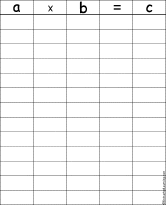Multiplication ChartWrite many equations in which axb=c, like 3x2=6. 1 Digit times 1 DigitMultiply two one-digit numbers.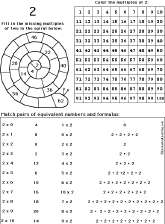Multiplying By Two: A Printable WorksheetFill in the missing multiples of two in the spiral, color the multiples of two in the grid of numbers from 1 to 100, and match the equivalent numbers and formulas. Go to the answers. Or go to a pdf version.Multiplying By Two, Part 2: A Printable WorksheetFill in the multiples of two in the circle, fill in the missing multiples of two in the circles, and fill in the missing numbers in the equations. Go to the answers. Or go to a pdf version.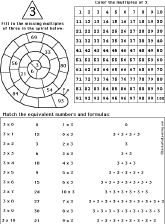Multiplying By Three: A Printable WorksheetFill in the missing multiples of three in the spiral, color the multiples of three in the grid of numbers from 1 to 100, and match the equivalent numbers and formulas. Go to the answers. Or go to a pdf version.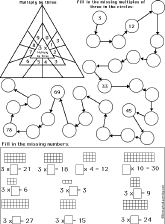Multiplying By Three, Part 2: A Printable WorksheetFill in the multiples of three in the triangle, fill in the missing multiples of three in the circles, and fill in the missing numbers in the equations. Go to the answers. Or go to a pdf version.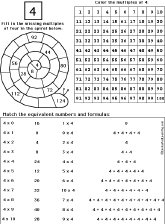Multiplying By Four: A Printable WorksheetFill in the missing multiples of four in the spiral, color the multiples of four in the grid of numbers from 1 to 100, and match the equivalent numbers and formulas. Go to the answers. Or go to a pdf version.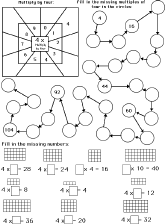Multiplying By Four, Part 2: A Printable WorksheetFill in the multiples of four in the square, fill in the missing multiples of four in the circles, and fill in the missing numbers in the equations. Go to the answers. Or go to a pdf version.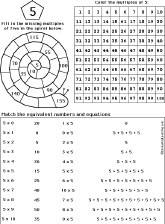Multiplying By Five: A Printable WorksheetFill in the missing multiples of five in the spiral, color the multiples of five in the grid of numbers from 1 to 100, and match the equivalent numbers and formulas. Go to the answers. Or go to a pdf version.Multiplying By Five, Part 2: A Printable WorksheetFill in the multiples of five in the pentagon, fill in the missing multiples of five in the circles, and fill in the missing numbers in the equations. Go to the answers. Or go to a pdf version.Multiplying By Six: A Printable WorksheetFill in the missing multiples of six in the spiral, color the multiples of six in the grid of numbers from 1 to 100, and match the equivalent numbers and formulas. Go to the answers. Or go to a pdf version.Multiplying By Six, Part 2: A Printable WorksheetFill in the multiples of six in the hexagon, fill in the missing multiples of six in the circles, and fill in the missing numbers in the equations. Go to the answers. Or go to a pdf version.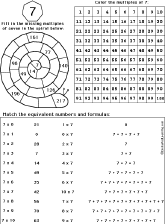Multiplying By Seven: A Printable WorksheetFill in the missing multiples of seven in the spiral, color the multiples of seven in the grid of numbers from 1 to 100, and match the equivalent numbers and formulas. Go to the answers. Or go to a pdf version.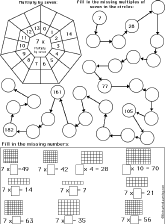Multiplying By Seven, Part 2: A Printable WorksheetFill in the multiples of seven in the septagon, fill in the missing multiples of seven in the circles, and fill in the missing numbers in the equations. Go to the answers. Or go to a pdf version.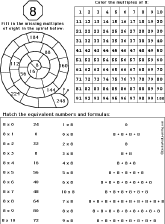Multiplying By Eight: A Printable WorksheetFill in the missing multiples of eight in the spiral, color the multiples of eight in the grid of numbers from 1 to 100, and match the equivalent numbers and formulas. Go to the answers. Or go to a pdf version.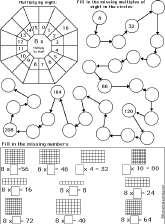Multiplying By Eight, Part 2: A Printable WorksheetFill in the multiples of eight in the octagon, fill in the missing multiples of eight in the circles, and fill in the missing numbers in the equations. Go to the answers. Or go to a pdf version.Multiplying By Nine: A Printable WorksheetFill in the missing multiples of nine in the spiral, color the multiples of nine in the grid of numbers from 1 to 100, and match the equivalent numbers and formulas. Go to the answers. Or go to a pdf version.Multiplying By Nine, Part 2: A Printable WorksheetFill in the multiples of nine in the nonagon, fill in the missing multiples of nine in the circles, and fill in the missing numbers in the equations. Go to the answers. Or go to a pdf version. 2 Digits times 1 DigitMultiply two-digit numbers times one-digit numbers.

 +, - EnchantedLearning.comMath x, ÷
 A B C D E F G H I J K L M N O P Q R S T U V W X Y Z
 Number Line Fractions Decimals Measurement Rounding Graphs

Enchanted Learning®
Over 35,000 Web Pages
Sample Pages for Prospective Subscribers, or click below

 Overview of Site What's New Enchanted Learning Home Monthly Activity Calendar Books to Print Site Index K-3 Crafts K-3 Themes Little ExplorersPicture dictionary PreK/K Activities Rebus Rhymes Stories Writing Cloze Activities Essay Topics Newspaper Writing Activities Parts of Speech Fiction The Test of Time iPhone app TapQuiz Maps - free iPhone Geography Game Biology Animal Printouts Biology Label Printouts Biomes Birds Butterflies Dinosaurs Food Chain Human Anatomy Mammals Plants Rainforests Sharks Whales Physical Sciences: K-12 Astronomy The Earth Geology Hurricanes Landforms Oceans Tsunami Volcano Languages Dutch French German Italian Japanese (Romaji) Portuguese Spanish Swedish Geography/History Explorers Flags Geography Inventors US History Other Topics Art and Artists Calendars College Finder Crafts Graphic Organizers Label Me! Printouts Math Music Word Wheels

## Enchanted Learning Search

 Search the Enchanted Learning website for: